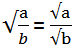# Operations On Real Numbers

You are already aware of the rational and irrational numbers. A rational number is a number which can be written as a ratio. Every rational number has a numerator and a denominator, that is, one integer divided by another integer. Whereas the numbers that cannot be expressed as a ratio of two integers are the irrational numbers, that is, the numbers that are not rational are irrational. The rational and irrational numbers together form the real numbers. In this article, we will discuss operations on real numbers – both rational and irrational.

## Operations on Real Numbers Rules

The following pointers are to be kept in mind when you deal with real numbers and mathematical operations on them:

• When the addition or subtraction operation is done on a rational and irrational number, the result is an irrational number.
• When the multiplication or division operation is done on a rational number with an irrational number, the result is an irrational number.
• When two irrational numbers are added, subtracted, multiplied or divided, the result may be a rational or an irrational number.

If a and b are positive real numbers, then we have,

• √ab = √a √b
•• (√a + √b)(√a – √b) = a – b
• (a + √b)(a – √b) = a2 – b
• (√a + √b)(√c + √d) = √ac + √ad + √bc + √bd
• (√a + √b)2 = a + 2√ab + b

## Video Lesson on Numbers### Operations on Real Numbers Examples

Example 1:

Solve (2√2 + 7√7) + (13√2 – 4√7).

Solution:

(2√2 + 7√7) + (13√2 – 4√7)

= (2√2 + 13√2) + (7√7 – 4√7)

= (2 + 13)√2 + (7-4)√7

= 15√2 + 3√7

Example 2:

Solve (7√7) x (- 4√7)

Solution:

(7√7) x (- 4√7)

= 7 x -4 x √7 x √7

= -28 x 7 = -196

Example 3:

Solve (8√21 / 4√7)

Solution:

(8√21 / 4√7)

= (8√7 x √3 / 4√7)

= 2 x √7 x √3 / √7 = 2√3

Example 4:

Solve (2√2 + 7√7)(2√2 – 7√7).

Solution:

(2√2 + 7√7) (2√2 – 7√7)

= (2√2)2 – (7√7)2

= 4 x 2 – 49 x 7

= 8 – 343 = -335

Example 5:

Solve (√2 + √7)(√3 – √11).

Solution:

(√2 + √7)(√3 – √11)

= √2√3 – √2√11 + √7√3 – √7√11

= √6 – √22 + √21 – √77